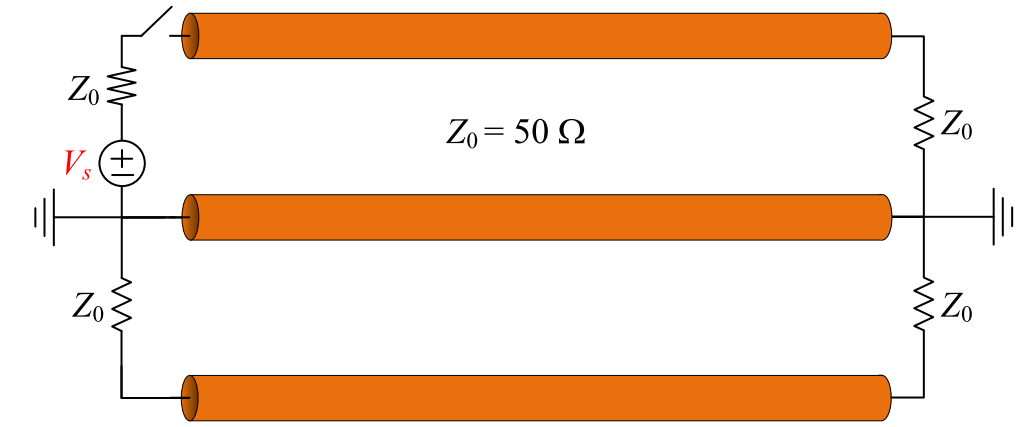Module 4.9 Crosstalk between Transmission LinesKb =       up*Kf =
 Press "Start" to update animation. NEXT       FEXT Step source     Pulse source

### Introduction:

 Show the near-end crosstalk (NEXT) and far-end crosstalk (FEXT) between two coupled transmission lines. One of the lines is active with source Vs and the other is quiet. Both lines have the same characteristic impedance of Z0 = 50 Ω and length of ℓ = 3 m.

### Parameters:

 CmL: the mutaul capacitance per length,in pF/m CL: the capacitance per length,in pF/m LmL: the mutaul inductance per length,in nH/m LL: the inductance per length,in nH/m TR: rise time up: phase velocity Kb = 0.25*(CmL/CL+LmL/LL) up*Kf = 0.5*(CmL/CL-LmL/LL) Plot 1: Source voltage Vs Plot 2: Blue curve: Near-end crosstalk along the quiet line t (v-z plot) Red curve: Far-end crosstalk along the quiet line t (v-z plot) Plot 3: Blue curve: Near-end crosstalk at z = 0 (v-t plot) Red curve: Far-end crosstalk at z = ℓ (v-t plot)